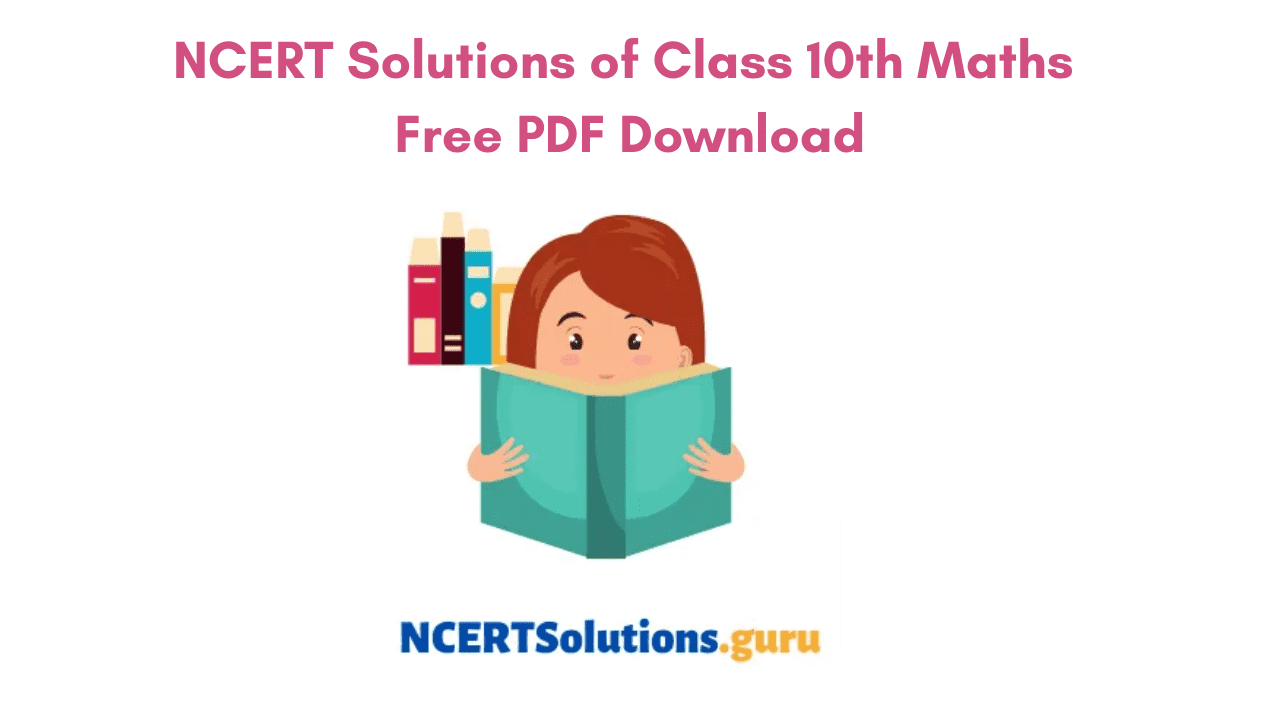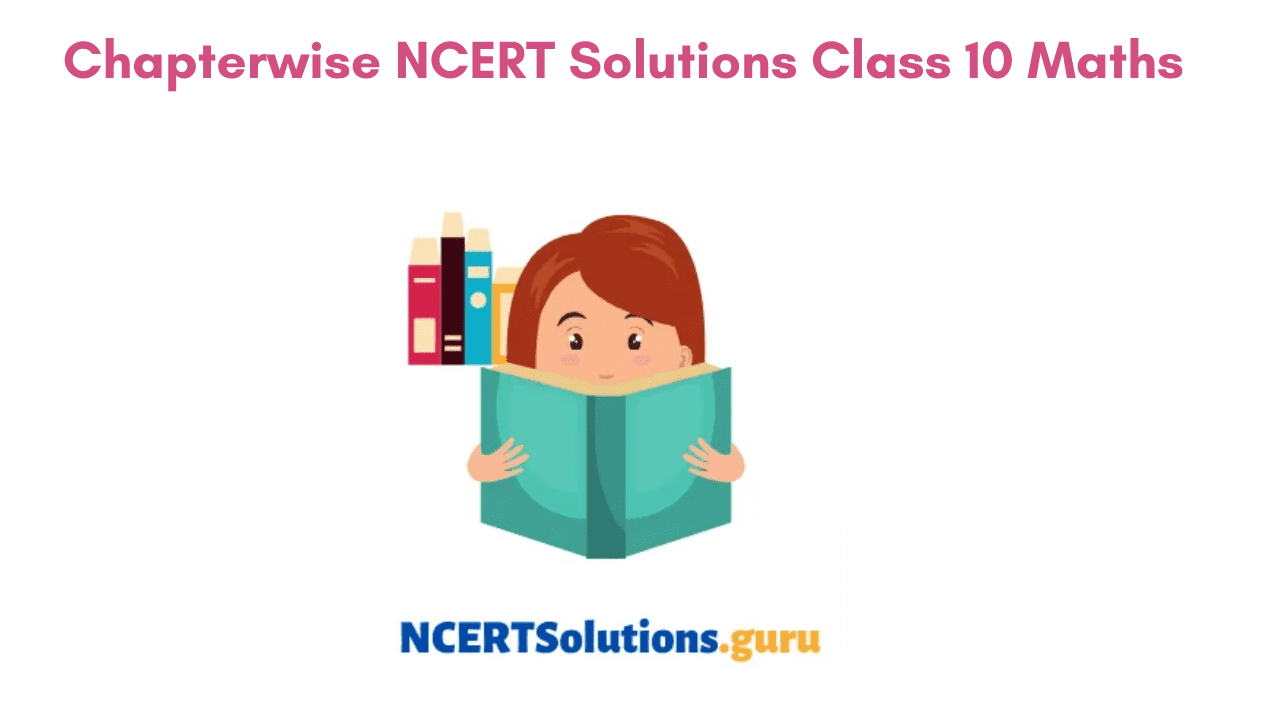# NCERT Solutions for Class 10 Maths in Hindi & English Medium | CBSE Class 10 Maths NCERT Textbook Solutions PDF

Download NCERT Solutions for Class 10 Maths in Hindi Medium for CBSE Board, UP Board (High School), MP Board, Gujrat Board, and other board’s students who are following NCERT Books. NCERT solutions for session 2021-22 is now available to download in PDF form. Download NCERT Solutions Apps for class 10 Hindi. According to the new CBSE Exam Pattern, MCQ Questions for Class 10 Maths Carries 20 Marks.

## CBSE Class 10th Maths NCERT Solutions in Hindi Medium and English Medium

उत्तर प्रदेश, मध्य प्रदेश, and गुजरात में भी NCERT की किताबें लगने के बाद हम लोग UP बोर्ड, MP बोर्ड, Gujrat Board के लिए भी अध्धयन सामग्री बना रहे हैं। अगर UP Board NCERT Solutions प्राप्त करने में विद्यार्थियों को किसी प्रकार की परेशानी हो रही है तो हमें Contact करें UP Board Secondary Education (High School) के लिए अध्ययन सामग्री, Sample Papers, Notes तथा पश्नों के हल यहाँ से प्राप्त करें।

NCERT Solutions For Class 10 Maths In Hindi and English Medium Pdf Download have been solved keeping in mind the guidelines issued by the CBSE. This has also been done in accordance with the marking scheme usually followed by the Board.### NCERT Solutions for Class 10 Maths Chapters Brief

Have an overview of the topics covered within the Class 10 Maths Syllabus chapterwise by referring to the below modules. Know the Unitwise Topics to be learned while preparing for the CBSE Class 10 Board Exam. They are as under

#### NCERT Solutions for Class 10 Maths Chapter 1 Real Numbers

In Class 10 Chapter 1 Real Numbers you will get to know more about real numbers and irrational numbers. The Chapter begins with Euclid’s Division Algorithm, What is Euclid’s Division Lemma and How to use it to calculate HCF of Two Numbers. Furthermore, you will know about rational numbers, irrational numbers and decimal expansion of rational numbers in detail.

#### NCERT Solutions for Class 10 Maths Chapter 2 Polynomials

In the Class 10th Maths Chapter 2 you will learn about the degree of the polynomial, linear polynomial, quadratic polynomial, etc. Topics discussed in this chapter are Zeros of a Polynomial Meaning, Relationship between Zeros and Coefficients of a Polynomial, Quadratic Polynomial, etc.

#### NCERT Solutions of Class 10 Maths Chapter 3 Pair of Linear Equations in Two Variables

In Ch 3 students will become familiar with the concept of pair of linear equations in two variables. The chapter deals with methods for solving linear equations, how to denote a situation graphically. Various methods like Algebraic Method, Elimination Method, Cross-Multiplication Method, Substitution Method, are explained in detail and questions are framed on the same in order to provide students with practice.

#### NCERT Solutions of Class 10 Maths Chapter 4 Quadratic Equations

Chapter 4 of 10th std Maths will give students knowledge on how to write the standard form of a quadratic equation. Methods like Completing the Square, Factorization for solving the Quadratic Equation are discussed. It further explains the topic of how to find the nature of the roots of a quadratic equation.

#### NCERT Solutions of Class 10 Maths Chapter 5 Arithmetic Progressions

In this chapter, you will come across a new topic called Arithmetic Progression, how to express a situation in the form of AP, finding the first term or difference in AP, checking if given series is in AP or not, etc. You will also know how to find the sum of first n terms in an Arithmetic Progression, find the nth term in an Arithmetic Progression, etc.

#### NCERT Solutions for Class 10 Maths Chapter 6 Triangles

In the CBSE Class 10 Maths Chapter 6, you will study the figures having the same shapes but not the same sizes. The chapter begins with the concepts of similar and congruent figures. It even describes the conditions for similarity of two triangles, theorems associated with similarity of triangles in detail. Furthermore, the Pythagoras Theorem and Converse of the Pythagoras Theorem are described.

#### NCERT Solutions of Class 10 Maths Chapter 7 Coordinate Geometry

In the Chapter of Coordinate Geometry, you will learn how to find the distance between two points whose coordinates are given, finding the area of a triangle given 3 points, etc. In addition, you will know how to find coordinates of a point that divides a line segment joining 2 given points in a given ratio, Distance Formula, Section Formula, etc.

#### NCERT Solutions of Class 10 Maths Chapter 8 Introduction to Trigonometry

In the CBSE Class 10 Maths Chapter 8, you will study ratios of the right triangle with respect to acute triangles. The chapter discusses trigonometric ratios of angles 00 and 900, how to find trigonometric ratios of specific angles involving these ratios namely trigonometric identities.

#### NCERT Solutions of Class 10 Maths Chapter 9 Some Applications of Trigonometry

In Class 10 Maths Ch 9 you will become familiar with the applications of trigonometry like geography,  construction of maps, navigation, determining the position of an island in relation to the longitudes and latitudes, etc. You can use trigonometry to determine the height of several objects without actually measuring them.

#### NCERT Solutions for Class 10 Maths Chapter 10 Circles

In Earlier Classes, you might have heard of various terms related to circles such as chord, segment, arc, etc. In CBSE Class 10 Maths students will learn about different situations that arise when a circle and line are given in a plane, the tangent of a circle, number of tangents from a point on a circle.

#### NCERT Solutions of Class 10 Maths Chapter 11 Constructions

In the NCERT Class 10 Maths Chapter 11 Constructions, students will get ideas of how to divide a line segment, how to construct tangents to a circle, etc. Various methods and steps of construction are explained so that you can have a clear idea of the topics.

#### NCERT Solutions of Class 10 Maths Chapter 12 Areas Related to Circles

Chapter 12 contains topics on perimeter and area of a circle, steps on how to find the area of a sector, segment of circular region, etc. In addition, you will know finding area of the combination of plane figures including circles or their parts, etc.  There are a total of 3 exercises in this chapter and all of them cover questions on the topics aforementioned.

#### NCERT Solutions of Class 10 Maths Chapter 13 Surface Areas and Volumes

Chapter 13 of CBSE Class 10 Maths includes concepts for finding the surface area of an object formed by combining any two solids such as cuboid, cone, cylinder, sphere, and hemisphere, etc. It even describes the concepts of volume, curved surface area, and total surface area of a frustum of a cone.

#### NCERT Solutions for Class 10 Maths Chapter 14 Statistics

The NCERT Solutions of Chapter 14 Statistics explains the numerical representation of ungrouped data to grouped data, how to find Mean, Median and Mode, etc. Concepts like Cumulative Frequency, How to draw Cumulative Frequency Curves are explained.

#### NCERT Solutions for Class 10 Maths Chapter 15 Probability

The last Chapter in CBSE Class 10 Maths Probability deals with topics like the difference between theoretical probability and experimental probability. Solve the exercise problems in the Class 10 Maths in Chapter Probability and get a grip of the topics.### CBSE Class 10th Marking Scheme 2021-22

CBSE Class 10 Maths Unitwise Marks Distribution for Term I

 Unit Unit Name Marks I Number systems 06 II Algebra 10 III Coordinate Geometry 06 IV Geometry 06 V Trigonometry 05 VI Mensuration 04 VII Statistics and Probability 03 Total 40 Internal Assessment 10 Total 50

CBSE Class 10 Maths Unitwise Marks Distribution for Term II

 Unit Unit Name Marks I Algebra (Cont.) 10 II Geometry (Cont.) 09 III Trigonometry (Cont.) 07 IV Mensuration (Cont.) 06 V Statistics and Probability (Cont.) 08 Total 40 Internal Assessment 10 Total 50

### FAQs on Class 10th Maths NCERT Solutions PDF

1. Where do I get the Class 10 Maths NCERT Solutions for All Chapters?

You can get the Class 10 Maths NCERT Solutions for All Chapters on our page.

2. How do NCERT Solutions of Class 10th Maths help me in my learning?

NCERT Solutions of Class 10 Maths helps in your overall learning and covers all the questions from NCERT Textbooks. Solve them regularly as a part of your practice and get a good hold of concepts.